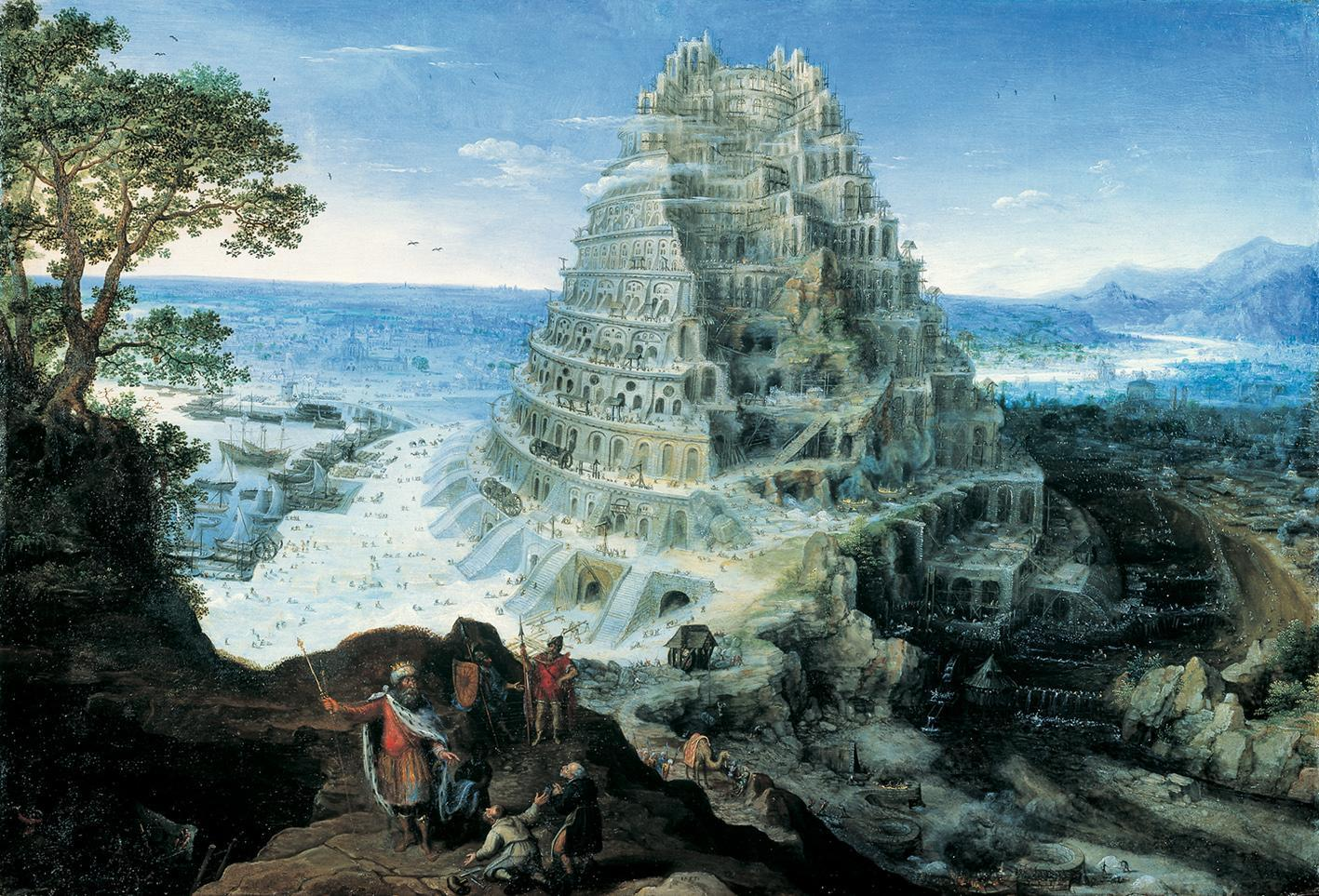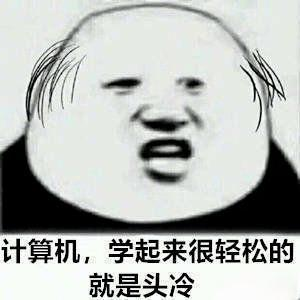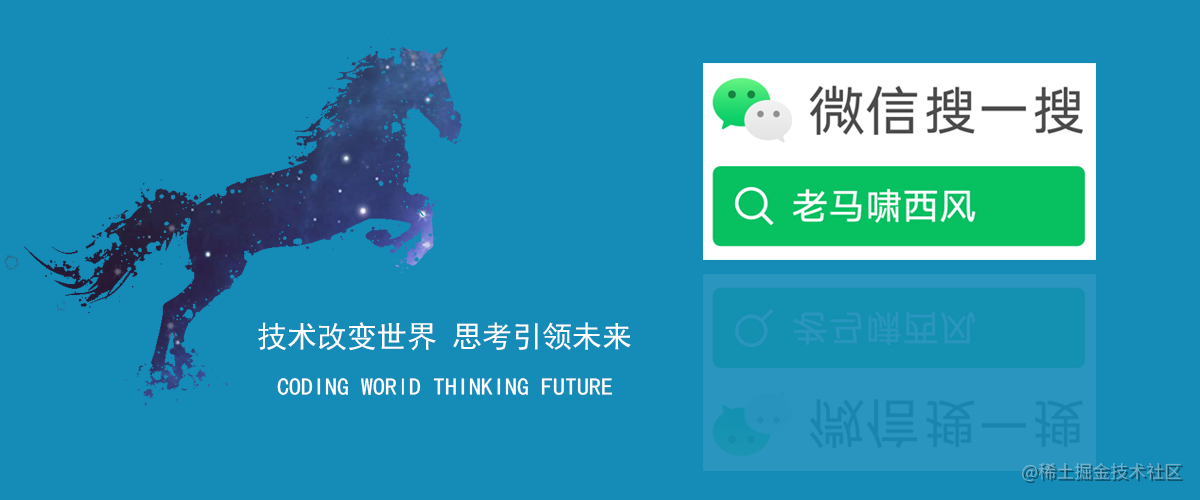# 不足之處

## 巴別塔

《聖經》中有關於巴別塔建造，最終人們因為語言問題而停工的故事​。``` 創11:6　“看哪！他們成為一樣的人民，都是一樣的言語，如今既作起這事來，以後他們所要作的事，就沒有不成就的了。

https://github.com/houbb/nlp-hanzi-similar/releases/tag/pythn

java 是一種語言，python 是一種語言。

# 拆字相似度

## 簡單的需求

``` 我的需求比較簡單。## 使用體驗

### maven 引入

```xml <dependency> <groupId>com.github.houbb</groupId> <artifactId>nlp-hanzi-similar</artifactId> <version>1.2.0</version> </dependency>```

### 使用

```java double rate1 = HanziSimilarHelper.similar('末', '未');```

https://github.com/houbb/nlp-hanzi-similar

# 寫在完結前

## 之後的計劃

NLP 的領域還有很多東西需要大家攻克，畢竟中文 NLP 才剛剛開始。# java 實現思路## 程式設計師的思維

（1）漢字的拆分實現

```java List<String> stringList = ChaiziHelper.chai(charWord.charAt(0));```

（2）相似的比較

A = {A1, A2, ..., Am}

B = {B1, B2, ..., Bm}

```java /* * 獲取拆分後對應的拆分字元 * @param charWord 字元 * @return 結果 / private char[] getSplitChars(String charWord) { List stringList = ChaiziHelper.chai(charWord.charAt(0));

``````// 這裡應該選擇哪一個是有講究的。此處為了簡單，預設選擇第一個。
String string = stringList.get(0);
return string.toCharArray();
``````

} ```

```java int minLen = Math.min(charsOne.length, charsTwo.length);

// 比較 double totalScore = 0.0; for(int i = 0; i < minLen; i++) { char iChar = charsOne[i]; String textChar = iChar+""; if(ArrayPrimitiveUtil.contains(charsTwo, iChar)) { //累加分數 } } ```

（3）拆分子集的權重

```java int textNumber = getNumber(textChar, similarContext);

double scoreOne = textNumber1.0 / numberOne * 1.0; double scoreTwo = textNumber1.0 / numberTwo * 1.0;

totalScore += (scoreOne + scoreTwo) / 2.0; ```

ps: 這裡的除以 2,是為了歸一化。保證最後的結果在 0-1 之間。

（4）筆畫數

```java private int getNumber(String text, IHanziSimilarContext similarContext) { Map<String, Integer> map = similarContext.bihuashuData().dataMap(); Integer number = map.get(text); if(number == null) { return 1; } return number; }```

## java 完整實現

```java /* * 拆字 * * @author 老馬嘯西風 * @since 1.0.0 / public class ChaiziSimilar implements IHanziSimilar {

``````@Override
public double similar(IHanziSimilarContext similarContext) {
String hanziOne = similarContext.charOne();
String hanziTwo = similarContext.charTwo();

int numberOne = getNumber(hanziOne, similarContext);
int numberTwo = getNumber(hanziTwo, similarContext);

// 拆分
char[] charsOne = getSplitChars(hanziOne);
char[] charsTwo = getSplitChars(hanziTwo);

int minLen = Math.min(charsOne.length, charsTwo.length);

// 比較
double totalScore = 0.0;
for(int i = 0; i <  minLen; i++) {
char iChar = charsOne[i];
String textChar = iChar+"";
if(ArrayPrimitiveUtil.contains(charsTwo, iChar)) {
int textNumber = getNumber(textChar, similarContext);

double scoreOne = textNumber*1.0 / numberOne * 1.0;
double scoreTwo = textNumber*1.0 / numberTwo * 1.0;

totalScore += (scoreOne + scoreTwo) / 2.0;
}
}

}

/**
* 獲取拆分後對應的拆分字元
* @param charWord 字元
* @return 結果
*/
private char[] getSplitChars(String charWord) {
List<String> stringList = ChaiziHelper.chai(charWord.charAt(0));

// 這裡應該選擇哪一個是有講究的。此處為了簡單，預設選擇第一個。
String string = stringList.get(0);

return string.toCharArray();
}

/**
* 獲取筆畫數
* @param text 文字
* @param similarContext 上下文
* @return 結果
*/
private int getNumber(String text, IHanziSimilarContext similarContext) {
Map<String, Integer> map = similarContext.bihuashuData().dataMap();

Integer number = map.get(text);
if(number == null) {
return 1;
}

return number;
}
``````

} ```

# 小結